基于YoloV3卫星图像的储油罐容积占用率研究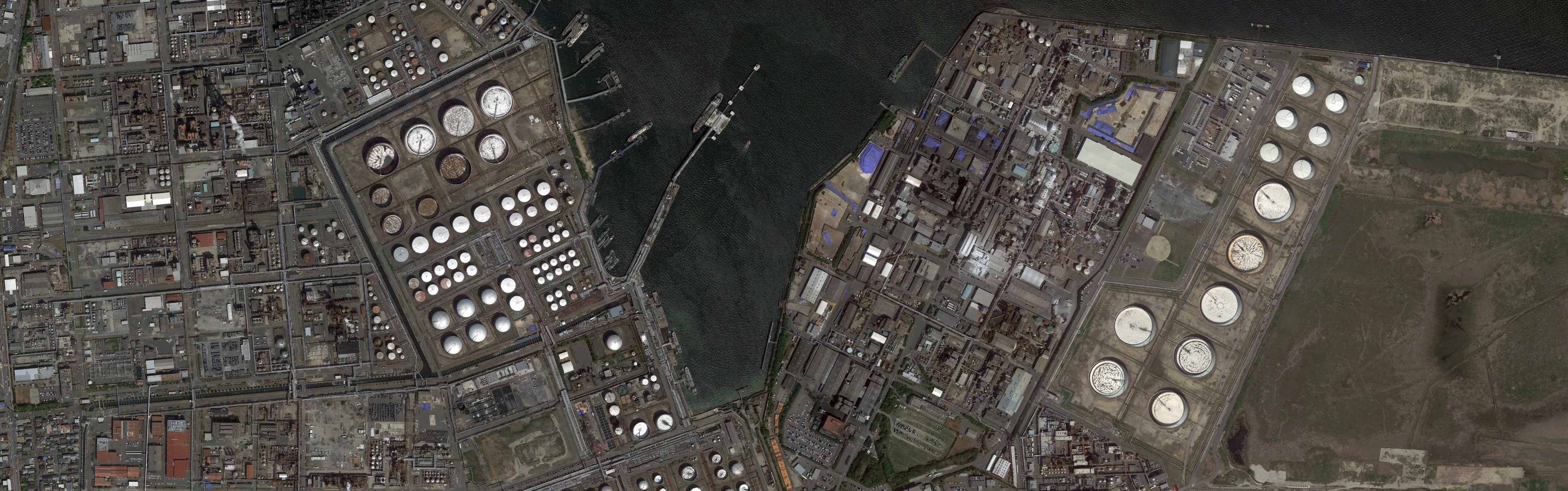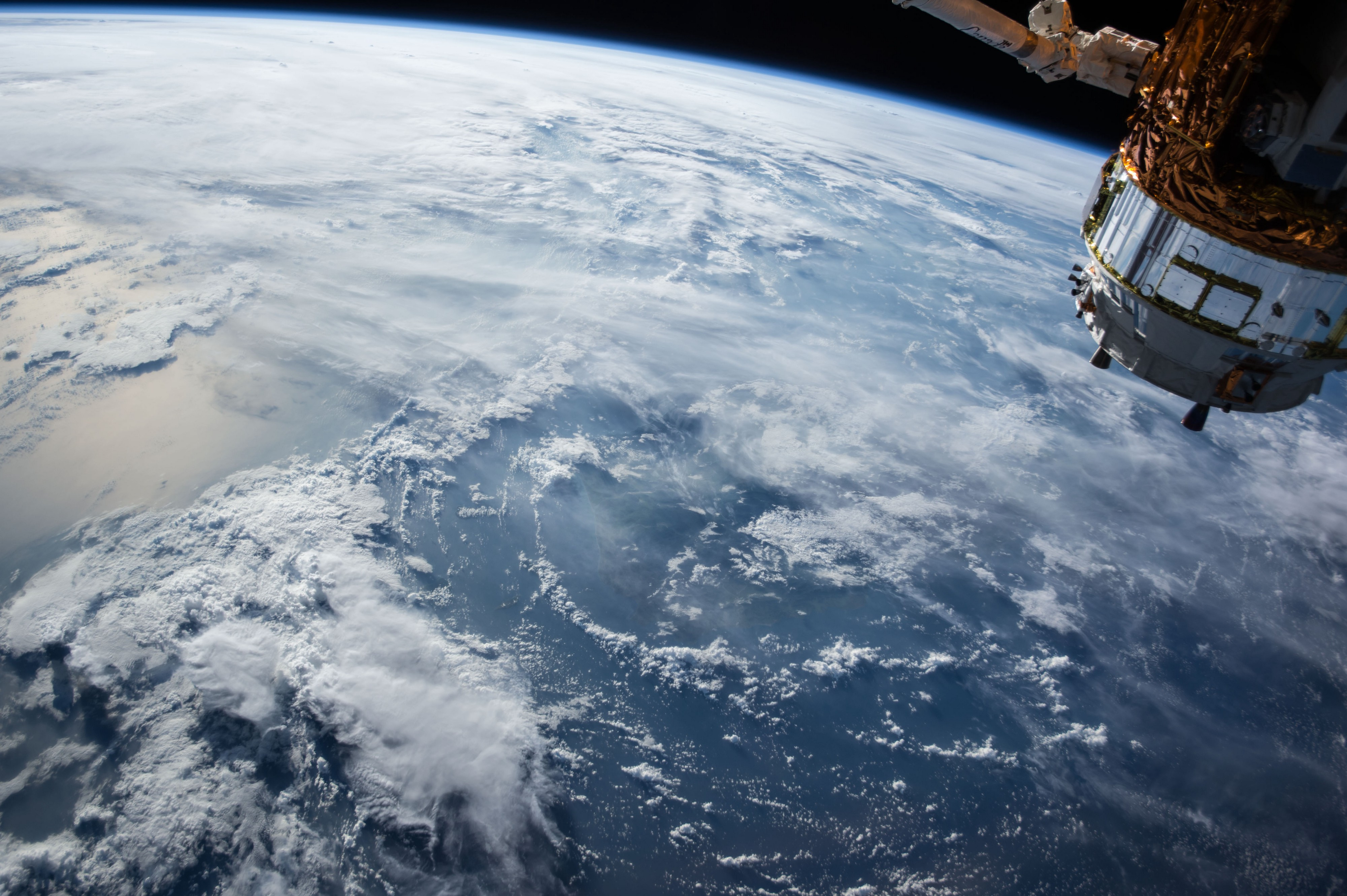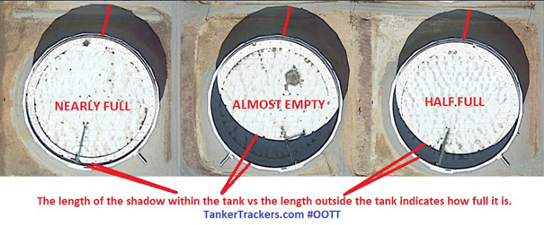(外部阴影)是指储罐的总高度，而储罐内的阴影(内部阴影)表示浮顶的深度。体积估计为1-(内部阴影区域/外部阴影区域)。

GitHub仓库

github.com/mdmub0587/O…

目录

1. 问题陈述、数据集和评估指标

2. 现有方法

3. 相关研究工作

4. 有用的博客和研究论文

5. 我们的贡献

6. 探索性数据分析(EDA)

7. 数据扩充

8. 数据预处理、扩充和TFRecords

9. 基于YoloV3的目标检测

10. 储量估算

11. 结果

12. 结论

13. 今后的工作

14. 参考引用

1.问题陈述、数据集和评估指标

数据集：

• large_images: 这是一个文件夹，包含100个卫星原始图像，每个大小为4800x4800。所有图像都以id_large.jpg格式命名。
• Image_patches: Image_patches目录包含从大图像生成的512x512大小的子图。每个大的图像被分割成100, 512x512大小的子图，两个轴上的子图之间有37个像素的重叠。生成图像子图的程序以id_row_column.jpg格式命名
• **labels.json:**它包含所有图像的标签。标签存储为字典列表，每个图像对应一个字典。不包含任何浮顶罐的图像将被标记为“skip”。边界框标签的格式为边界框四个角的(x，y)坐标。
• labels_coco.json: 它包含与前一个文件相同的标签，转换为COCO标签格式。在这里，边界框的格式为[x_min, y_min, width, height].
• **large_image_data.csv:**它包含有关大型图像文件的元数据，包括每个图像的中心坐标和海拔高度。

2.现有方法

Karl Keyer 在他的存储库中使用RetinaNet来完成储油罐探测任务。他从头开始创建模型，并将生成的锚框应用于该数据集。这使得浮顶罐的平均精度(AP)达到76.3%。然后他应用阴影增强和像素阈值法来计算它的体积。

4.有用的博客和研究论文

A Gentle Introduction to Object Recognition With Deep Learning  :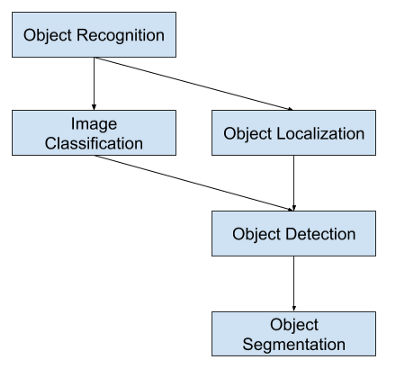Selective Search for Object Recognition :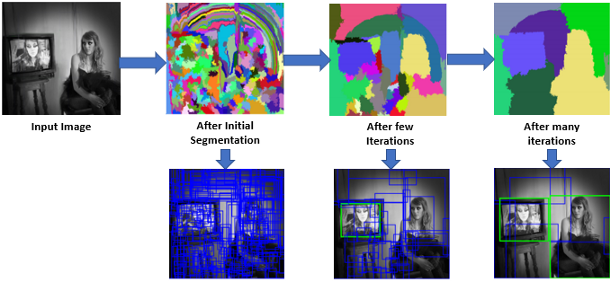Region Proposal Network — A detailed view：

RPN(Region-proposition Network)由于其比传统的选择性搜索算法更快而被广泛地应用于目标定位。它从特征地图中学习目标的最佳位置，就像CNN从特征图中学习分类一样。

Faster R-CNN: Towards Real-Time Object Detection with Region Proposal Networks：

Faster R-CNN模型解决了前两个相关模型(R-CNN和Fast R-CNN)的所有问题，并使用RPN作为区域建议生成器。它的架构与Fast R-CNN完全相同，只是它使用了RPN而不是选择性搜索，这使得它比Fast R-CNN快34倍。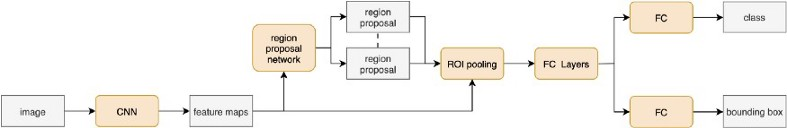Real-time Object Detection with YOLO, YOLOv2, and now YOLOv3 ：

youtu.be/Cgxsv1riJhI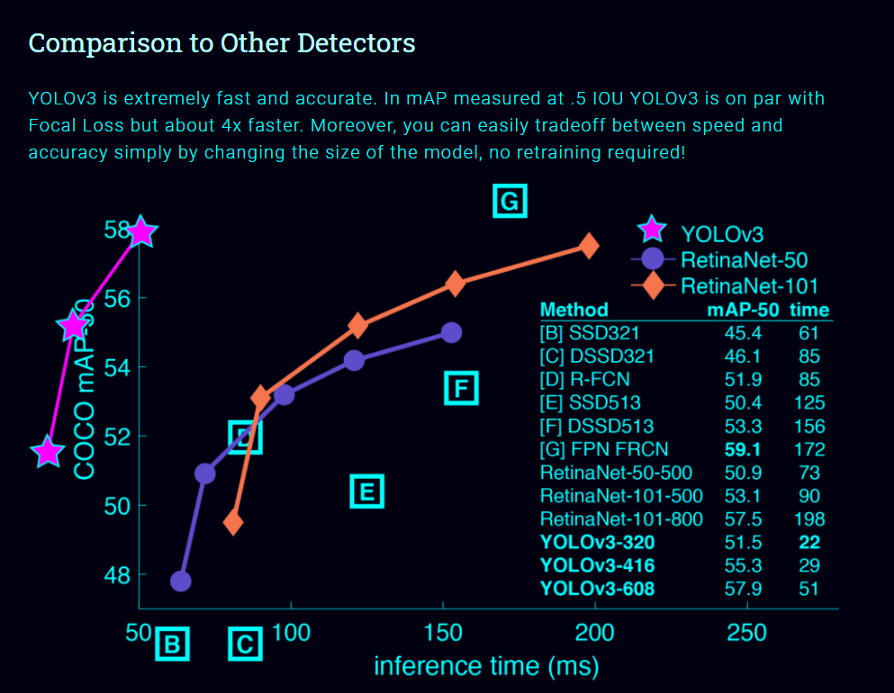• 单神经网络模型(即分类和定位任务都将从同一个模型中执行)：以一张照片作为输入，直接预测每个边界框的边界框和类标签，这意味着它只看一次图像。

• 由于它对整个图像而不是图像的一部分执行卷积，因此它产生的背景错误非常少。

• YOLO学习对象的一般化表示。在对自然图像进行训练和艺术品测试时，YOLO的性能远远超过DPM和R-CNN等顶级检测方法。由于YOLO具有高度的通用性，所以当应用于新的域或意外的输入时，它不太可能崩溃。

• 如果你仔细看一下yolov2论文的标题，那就是“YOLO9000: Better, Faster, Stronger”。yolov3比yolov2好得多吗？好吧，答案是肯定的，它更好，但不是更快更强，因为体系的复杂性增加了。

• Yolov2使用了19层DarkNet架构，没有任何残差块、skip连接和上采样，因此它很难检测到小对象。然而，在Yolov3中，这些特性被添加，并且使用了在Imagenet上训练的53层DarkNet网络。除此之外，还堆积了53个卷积层，形成了106个卷积层结构。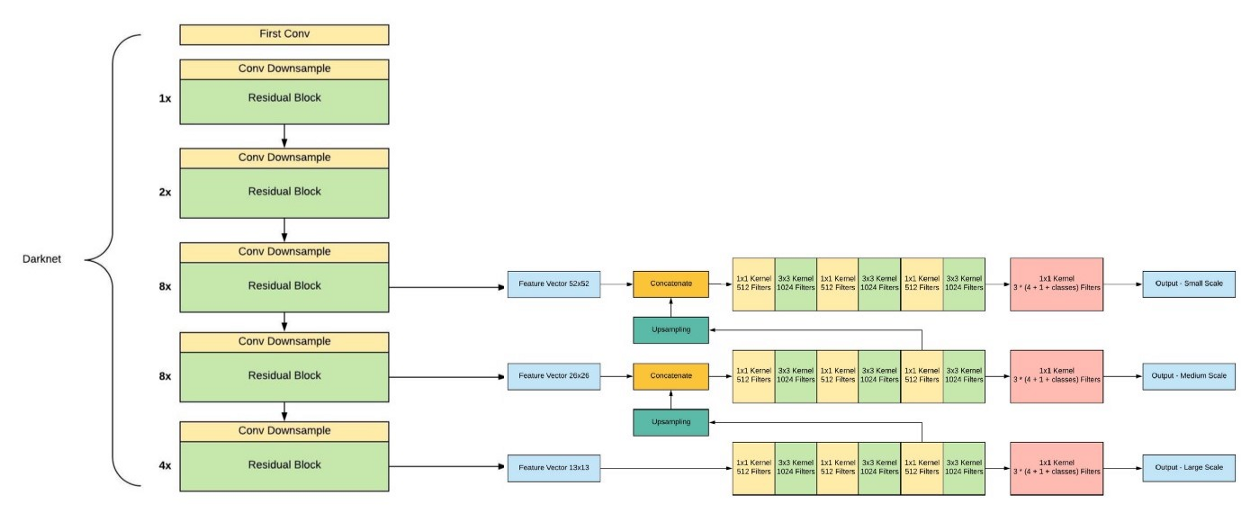• Yolov3在三种不同的尺度上进行预测，首先是大对象的13X13网格，其次是中等对象的26X26网格，最后是小对象的52X52网格。

• YoloV3总共使用9个锚箱，每个标度3个。用K均值聚类法选出最佳锚盒。

• Yolov3现在对图像中检测到的对象执行多标签分类。通过logistic回归预测对象置信度和类预测。

5.我们的贡献

阴影提取和体积估计：

www.kaggle.com/towardsentr…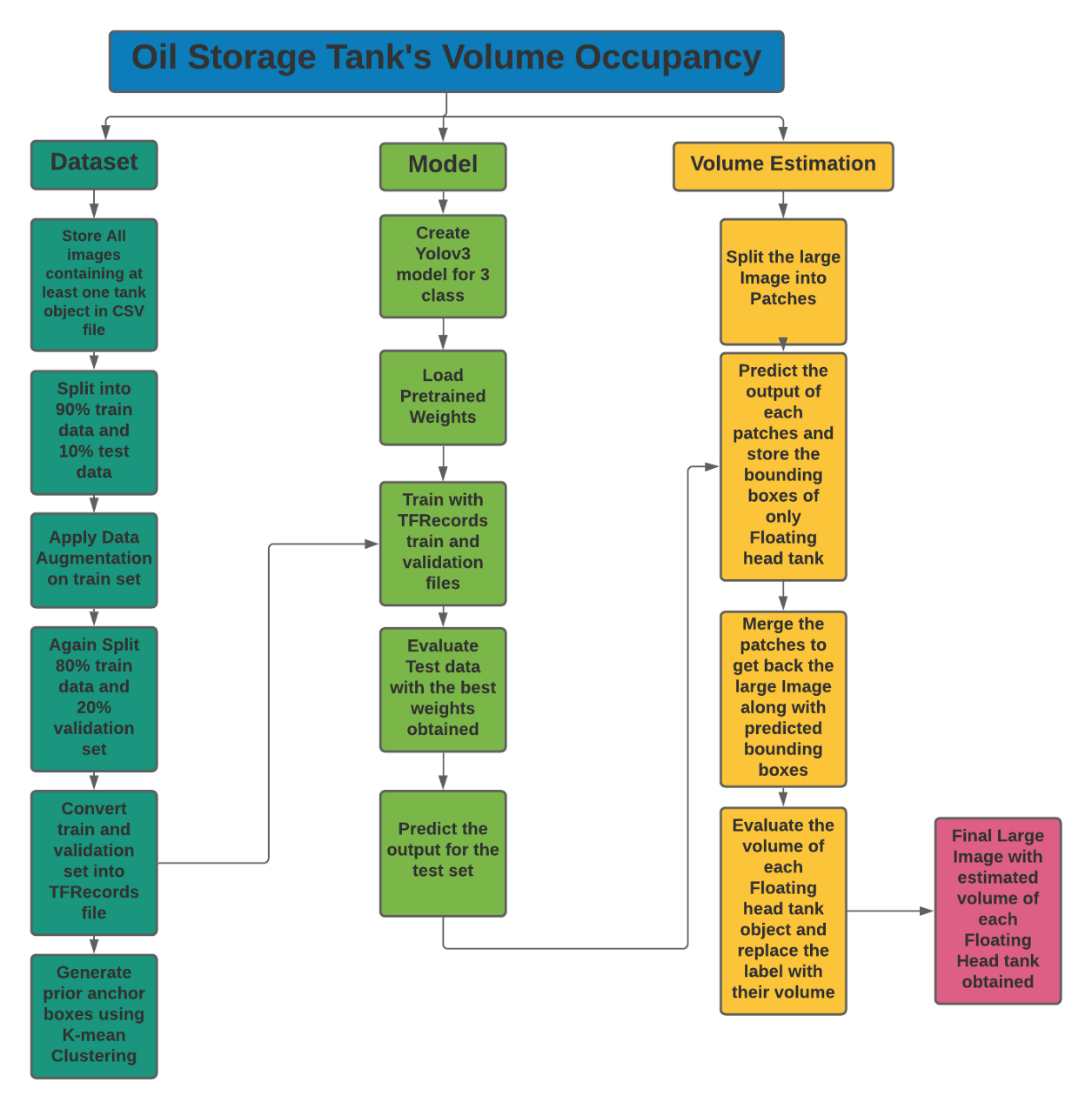6.探索性数据分析(EDA)

探索Labels.json文件：

print('Number of Images: ',len(json_labels))
json_labels[25:30]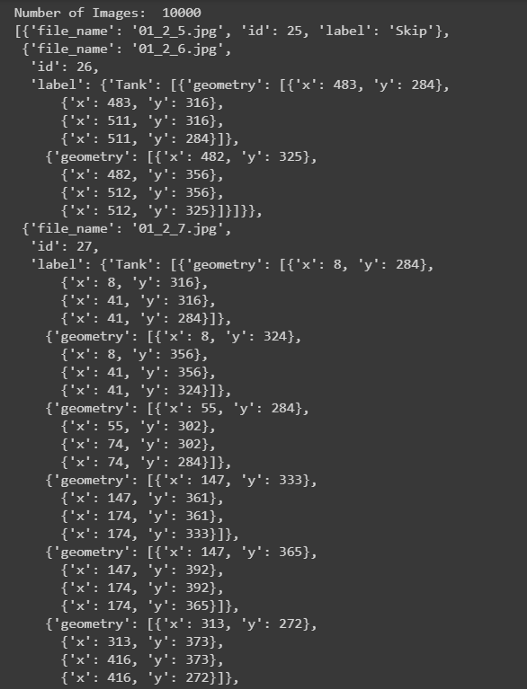计数：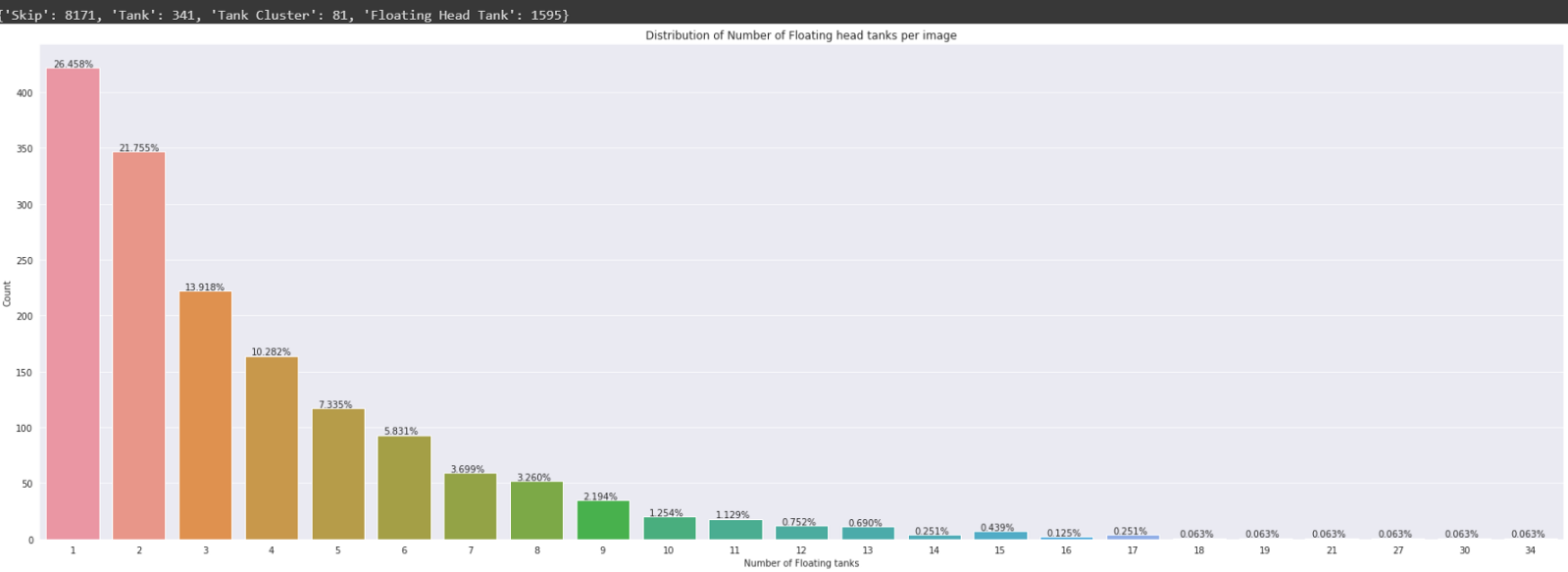探索labels_coco.json文件：

print('Number of Floating tanks: ',len(json_labels_coco['annotations']))
no_unique_img_id = set()
for ann in json_labels_coco['annotations']:
print('Number of Images that contains Floating head tank: ', len(no_unique_img_id))
json_labels_coco['annotations'][:8]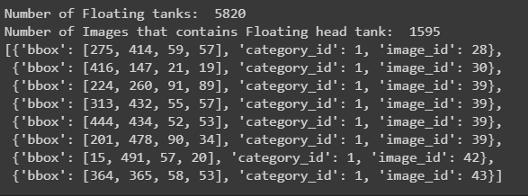打印边界框：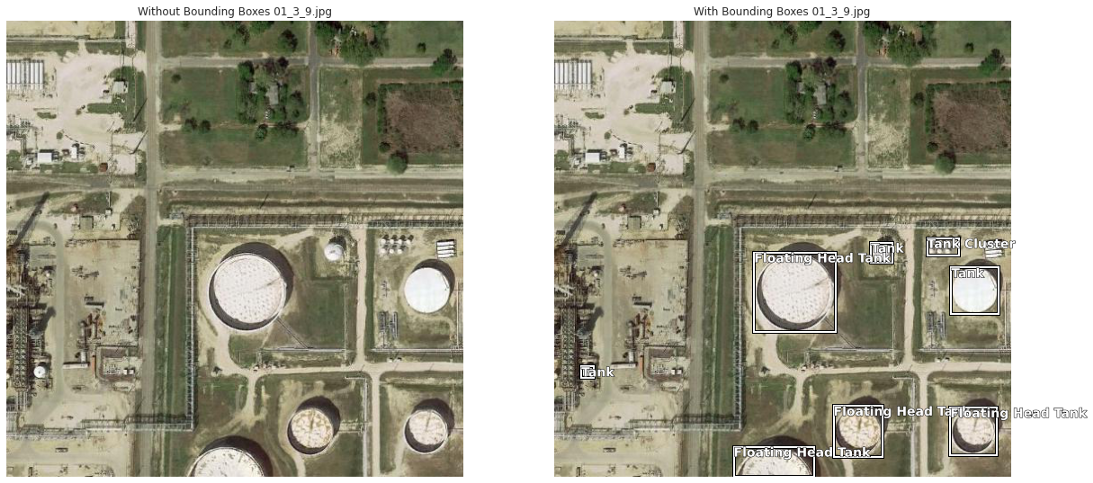1. Tank(T 油罐)

2. Tank Cluster(TC 油罐组),

8.数据预处理、扩充和TFRecords

数据预处理：

def conv_bbox(box_dict):
"""
input: box_dict-> 字典中有4个角点
Function: 获取左上方和右下方的点
output: tuple(ymin, xmin, ymax, xmax)
"""
xs = np.array(list(set([i['x'] for i in box_dict])))
ys = np.array(list(set([i['y'] for i in box_dict])))
x_min = xs.min()
x_max = xs.max()
y_min = ys.min()
y_max = ys.max()

return y_min, x_min, y_max, x_max

CSV文件将如下所示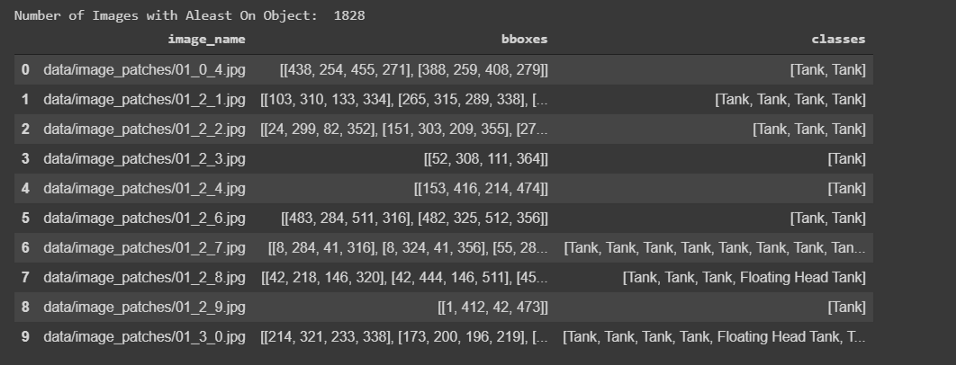# 训练和测试划分
df_train, df_test= model_selection.train_test_split(
df, #CSV文件注释
test_size=0.1,
random_state=42,
shuffle=True,
)
df_train.shape, df_test.shape

数据扩充：

blog.paperspace.com/data-augmen…

1. 水平翻转

2. 旋转90度

3. 旋转180度

4. 旋转270度

5. 水平翻转和90度旋转

6. 水平翻转和180度旋转

7. 水平翻转和270度旋转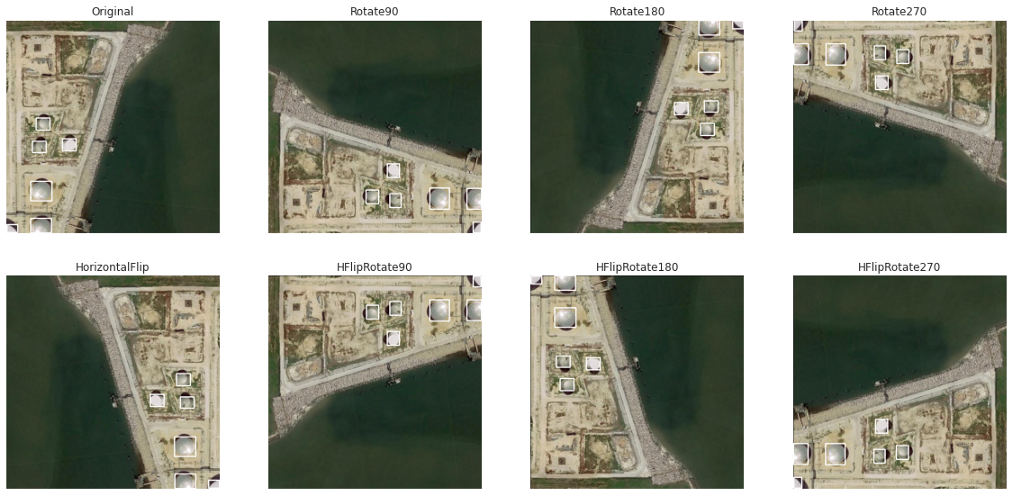TFRecords：

TFRecords是TensorFlow自己的二进制存储格式。当数据集太大时，它通常很有用。它以二进制格式存储数据，并对训练模型的性能产生显著影响。二进制数据复制所需的时间更少，而且由于在训练时只加载了一个batch数据，所以占用的空间也更少。你可以在下面的博客中找到它的详细描述。

medium.com/mostly-ai/t…

www.tensorflow.org/tutorials/l…

9.基于YoloV3的目标检测

训练：

def create_model():
tf.keras.backend.clear_session()
pret_model = YoloV3(size, channels, classes=80)

model = YoloV3(size, channels, classes=3)
model.get_layer('yolo_darknet').set_weights(
pret_model.get_layer('yolo_darknet').get_weights())

freeze_all(model.get_layer('yolo_darknet'))
print('Frozen DarkNet layers')
return model

model = create_model()
model.summary()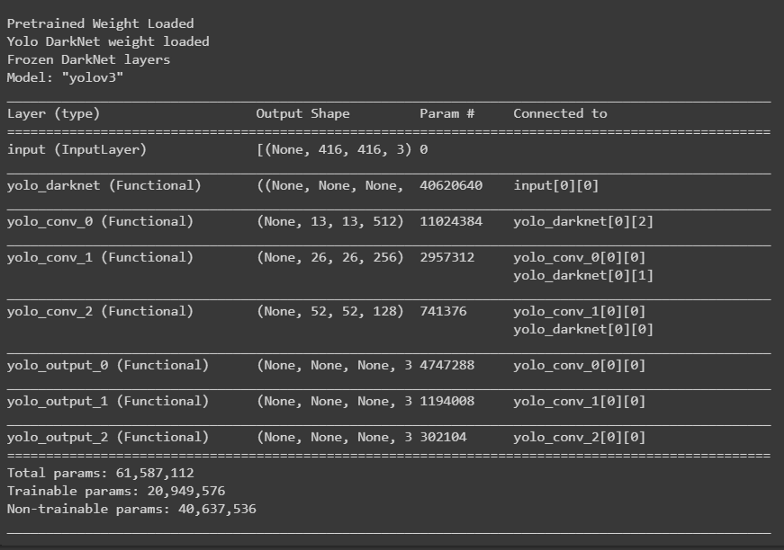tf.keras.backend.clear_session()
epochs = 100
learning_rate=1e-3

optimizer = get_optimizer(
learning_rate=1e-3,
decay_type='cosine',
decay_steps=10*600
)

model = create_model()
model.compile(optimizer=optimizer, loss=loss)

# Tensorbaord
! rm -rf ./logs/
logdir = os.path.join("logs", datetime.datetime.now().strftime("%Y%m%d-%H%M%S"))
%tensorboard --logdir \$logdir
tensorboard_callback = tf.keras.callbacks.TensorBoard(logdir, histogram_freq=1)

callbacks = [
EarlyStopping(monitor='val_loss', min_delta=0, patience=15, verbose=1),
ModelCheckpoint('Weights/Best_weight.hdf5', verbose=1, save_best_only=True),
tensorboard_callback,
]

history = model.fit(train_dataset,
epochs=epochs,
callbacks=callbacks,
validation_data=valid_dataset)
model.save('Weights/Last_weight.hdf5')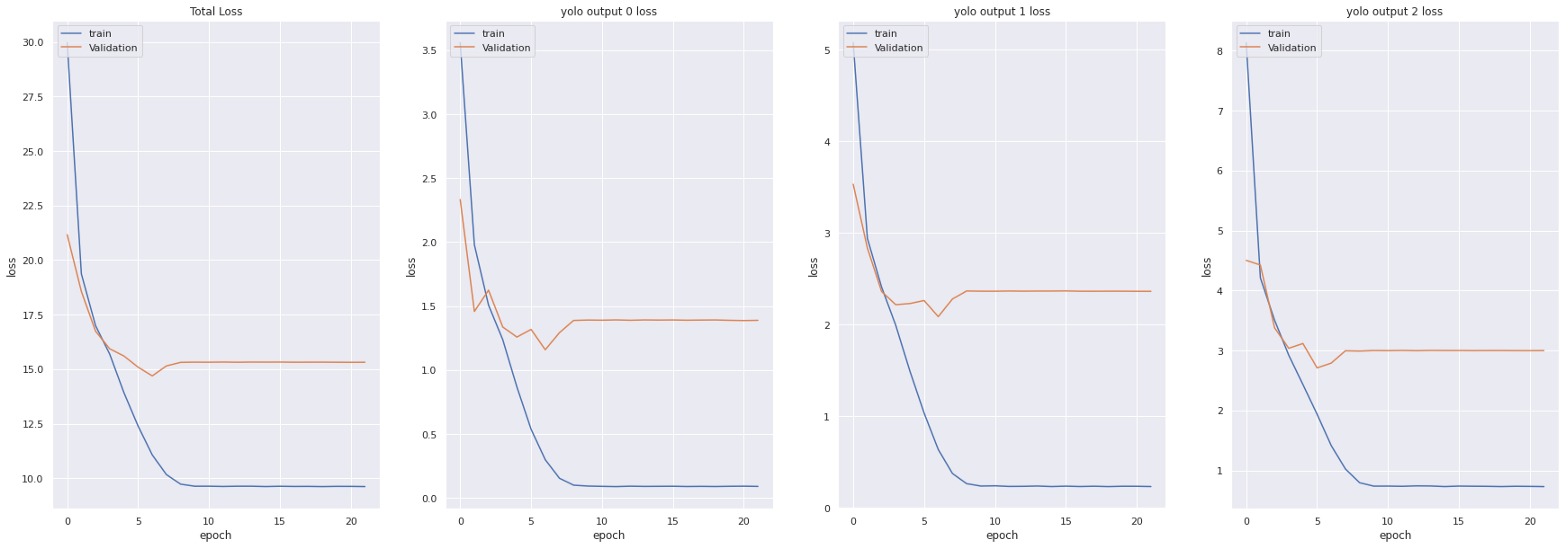YOLO损失函数：

Yolov3模型训练中所用的损失函数相当复杂。Yolo在三个不同的尺度上计算三个不同的损失，并对反向传播进行总结(正如你在上面的代码单元中看到的，最终损失是三个不同损失的列表)。每个loss都通过4个子函数计算定位损失和分类损失。

1. 中心(x，y) 的MSE损失.
2. 边界框的宽度和高度的均方误差(MSE)
3. 边界盒的二元交叉熵得分与无目标得分
4. 边界盒多类预测的二元交叉熵或稀疏范畴交叉熵Yolov2中的最后三项是平方误差，而在Yolov3中，它们被交叉熵误差项所取代。换句话说，Yolov3中的对象置信度和类预测现在通过logistic回归进行预测。

def YoloLoss(anchors, classes=3, ignore_thresh=0.5):
def yolo_loss(y_true, y_pred):
# 1. 转换所有预测输出
# y_pred: (batch_size, grid, grid, anchors, (x, y, w, h, obj, ...cls))
pred_box, pred_obj, pred_class, pred_xywh = yolo_boxes(
y_pred, anchors, classes)
# predicted (tx, ty, tw, th)
pred_xy = pred_xywh[..., 0:2] #x,y of last channel
pred_wh = pred_xywh[..., 2:4] #w,h of last channel

# 2. 转换所有真实输出
# y_true: (batch_size, grid, grid, anchors, (x1, y1, x2, y2, obj, cls))
true_box, true_obj, true_class_idx = tf.split(
y_true, (4, 1, 1), axis=-1)

#转换 x1, y1, x2, y2 to x, y, w, h
# x,y = (x2 - x1)/2, (y2-y1)/2
# w, h = (x2- x1), (y2 - y1)
true_xy = (true_box[..., 0:2] + true_box[..., 2:4]) / 2
true_wh = true_box[..., 2:4] - true_box[..., 0:2]

# 小盒子要更高权重
#shape-> (batch_size, grid, grid, anchors)
box_loss_scale = 2 - true_wh[..., 0] * true_wh[..., 1]

# 3. 对pred box方程反向
# 把 (bx, by, bw, bh) 变为 (tx, ty, tw, th)
grid_size = tf.shape(y_true)
grid = tf.meshgrid(tf.range(grid_size), tf.range(grid_size))
grid = tf.expand_dims(tf.stack(grid, axis=-1), axis=2)
true_xy = true_xy * tf.cast(grid_size, tf.float32) - tf.cast(grid, tf.float32)
true_wh = tf.math.log(true_wh / anchors)
# 可能有些格的true_wh是0, 用锚点划分可能导致inf或nan
true_wh = tf.where(tf.logical_or(tf.math.is_inf(true_wh),
tf.math.is_nan(true_wh)),
tf.zeros_like(true_wh), true_wh)

# 4. 计算所有掩码
#从张量的形状中去除尺寸为1的维度。
#当iou超过临界值时，忽略假正例
#best_iou: (batch_size, grid, grid, anchors)
best_iou = tf.map_fn(
x, tf.cast(x, tf.bool))), axis=-1),
tf.float32)
ignore_mask = tf.cast(best_iou < ignore_thresh, tf.float32)

# 5.计算所有损失
xy_loss = obj_mask * box_loss_scale * \
tf.reduce_sum(tf.square(true_xy - pred_xy), axis=-1)
wh_loss = obj_mask * box_loss_scale * \
tf.reduce_sum(tf.square(true_wh - pred_wh), axis=-1)
obj_loss = binary_crossentropy(true_obj, pred_obj)
obj_loss = obj_mask * obj_loss + \
#TODO:使用binary_crossentropy代替
true_class_idx, pred_class)

# 6. 在(batch, gridx, gridy, anchors)求和得到 => (batch, 1)
xy_loss = tf.reduce_sum(xy_loss, axis=(1, 2, 3))
wh_loss = tf.reduce_sum(wh_loss, axis=(1, 2, 3))
obj_loss = tf.reduce_sum(obj_loss, axis=(1, 2, 3))
class_loss = tf.reduce_sum(class_loss, axis=(1, 2, 3))

return xy_loss + wh_loss + obj_loss + class_loss
return yolo_loss

get_mAP(model, 'data/test.csv')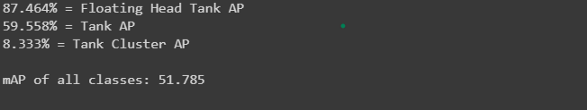get_mAP(model, 'data/train.csv')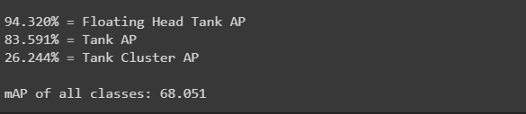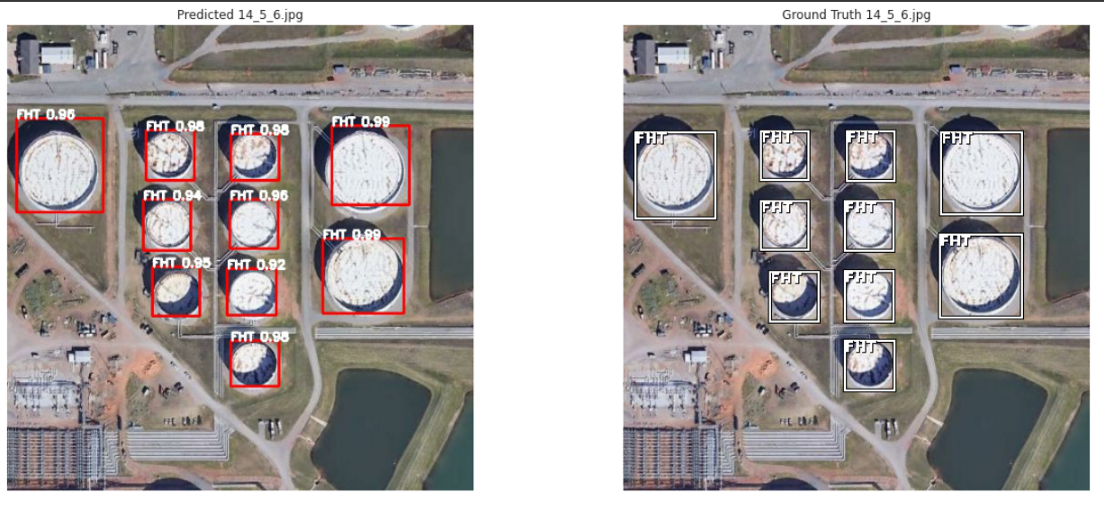10.储量估算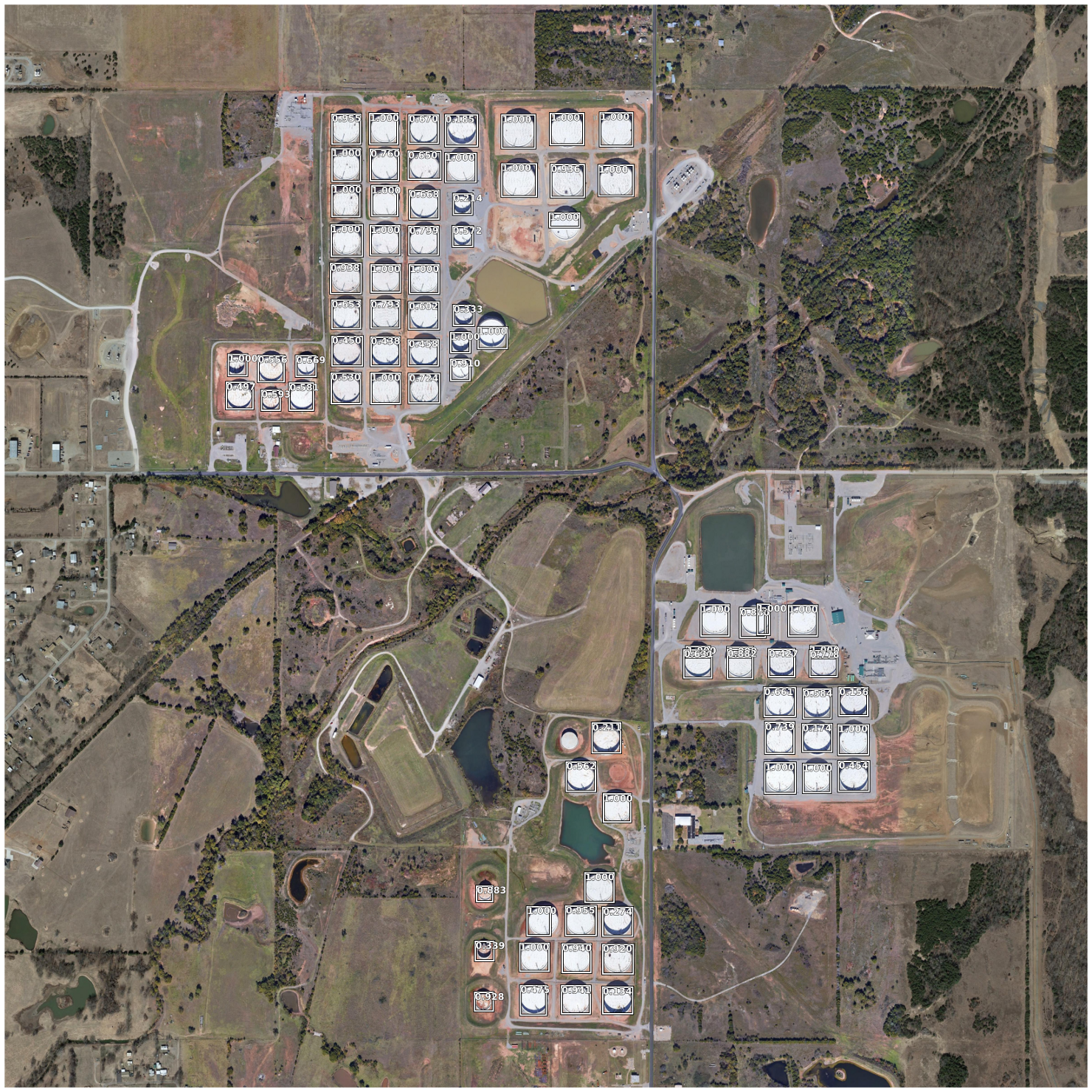12.结论

• 只需有限的图像就可以得到相当好的结果。

• 数据扩充工作得很到位。

• 在本例中，与RetinaNet模型的现有方法相比，yolov3表现得很好。

13.今后的工作

• 浮顶罐的AP值为87.4%，得分较高。然而，我们可以尝试在更大程度上提高分数。

• 我们将尝试生成的更多数据来训练这个模型。

• 我们将尝试训练另一个更精确的模型，如yolov4，yolov5(非官方)。

14.参考引用

 Oil-Tank-Volume-Estimation, by Karl Heyer, Nov 2019. （github.com/kheyer/Oil-…

 Estimating the Volume of Oil Tanks Based on High-Resolution Remote Sensing Images by Tong Wang, Ying Li, Shengtao Yu, and Yu Liu, April 2019.（www.researchgate.net/publication…

 A Beginner’s Guide To Calculating Oil Storage Tank Occupancy With Help Of Satellite Imagery by TankerTrackers.com, Sep 2017.（medium.com/planet-stor…

 A Gentle Introduction to Object Recognition With Deep Learning by machinelearningmastery.com/, May 2019.（machinelearningmastery.com/object-reco…

 Selective Search for Object Recognition by J.R.R. Uijlings at el. 2012（www.huppelen.nl/publication…

 Region Proposal Network — A detailed view by Sambasivarao. K, Dec 2019（towardsdatascience.com/region-prop…

 Faster R-CNN: Towards Real-Time Object Detection with Region Proposal Networks by Ross Girshick et al. Jan 2016.（arxiv.org/abs/1506.01…

 Real-time Object Detection with YOLO, YOLOv2 and now YOLOv3 by Joseph Redmon, 2015–2018 （arxiv.org/abs/1506.02…

sklearn机器学习中文官方文档： sklearn123.com/